• R语言多元Logistic回归 应用案例 多元Logistic回归 如何进行多重逻辑回归 可以使用阶梯函数通过逐步过程确定多重逻辑回归。此函数选择模型以最小化AIC，而不是像手册中的SAS示例那样根据p值。另请注意，在此...
原文链接：http://tecdat.cn/?p=2640

原文出处：拓端数据部落公众号

可以使用逐步回归过程确定多元逻辑回归。此函数选择模型以最小化AIC。

如何进行多元逻辑回归

可以使用step函数通过逐步回归过程确定多元逻辑回归。此函数选择模型以最小化AIC。

通常建议不要盲目地遵循逐步回归程序，而是要使用拟合统计（AIC，AICc，BIC）比较模型，或者根据生物学或科学上合理的可用变量建立模型。

多元相关是研究潜在自变量之间关系的一种工具。例如，如果两个独立变量彼此相关，可能在最终模型中都不需要这两个变量，但可能有理由选择一个变量而不是另一个变量。

多元相关

创建数值变量的数据框



Data.num $Status = as.numeric（Data.num$ Status）

Data.num $Length = as.numeric（Data.num$ Length）

Data.num $Migr = as.numeric（Data.num$ Migr）

Data.num $Insect = as.numeric（Data.num$ Insect）

Data.num $Diet = as.numeric（Data.num$ Diet）

Data.num $Broods = as.numeric（Data.num$ Broods）

Data。 num $Wood = as.numeric（Data.num$ Wood）

Data.num $Upland = as.numeric（Data.num$ Upland）

Data.num $Water = as.numeric（Data.num$ Water）

Data.num $Release = as.numeric（Data.num$ Release）

Data.num $Indiv = as.numeric（Data.num$ Indiv）

###检查新数据框

headtail（Data.num）

1 1 1520 9600.0 1.21 1 12 2 6.0 1 0 0 1 6 29

2 1 1250 5000.0 0.56 1 0 1 6.0 1 0 0 1 10 85

3 1 870 3360.0 0.07 1 0 1 4.0 1 0 0 1 3 8

77 0 170 31.0 0.55 3 12 2 4.0 NA 1 0 0 1 2

78 0 210 36.9 2.00 2 8 2 3.7 1 0 0 1 1 2

79 0 225 106.5 1.20 2 12 2 4.8 2 0 0 0 1 2

###检查变量之间的相关性

###这里使用了Spearman相关性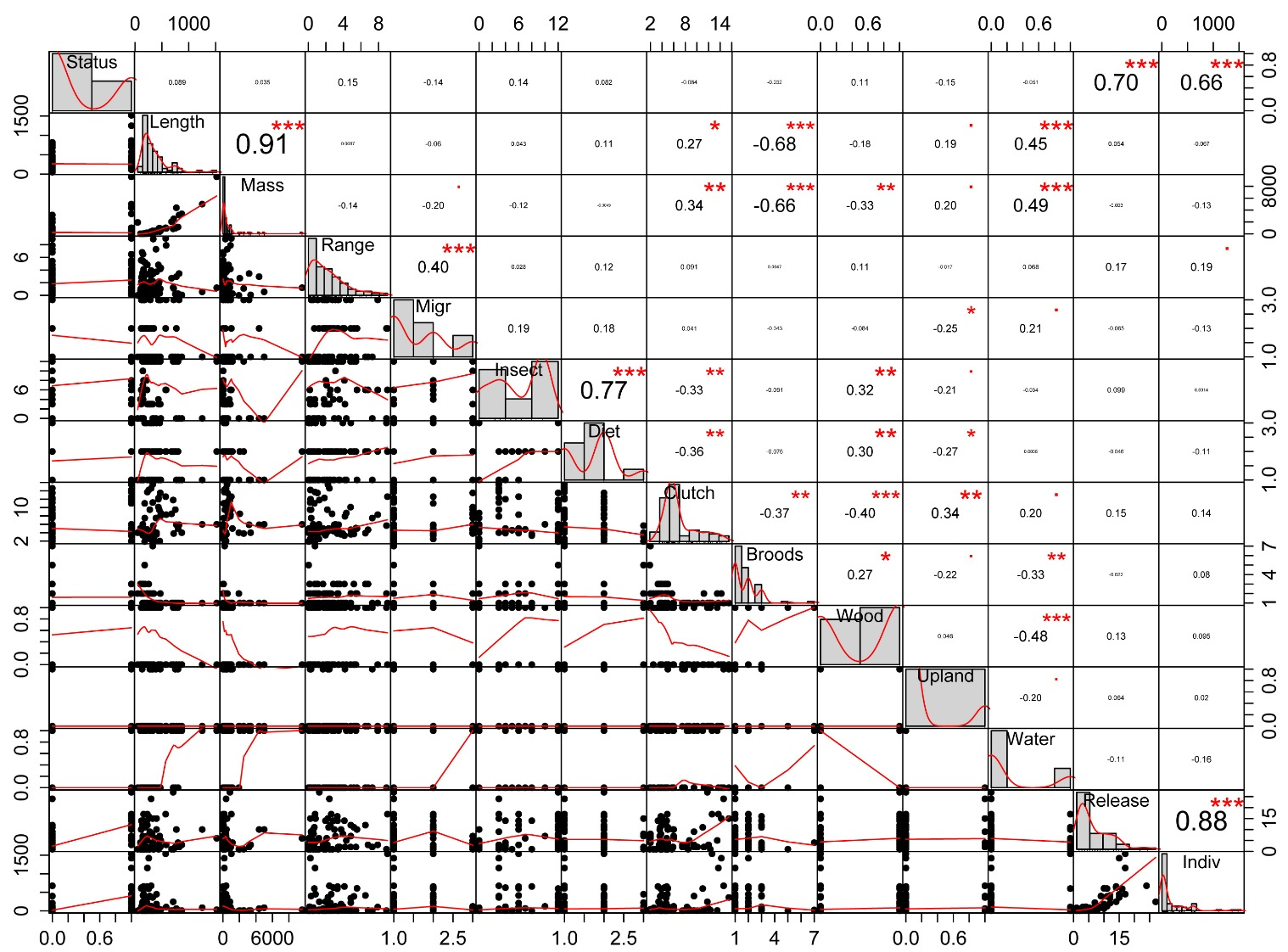多元逻辑回归的例子

在此示例中，数据包含缺失值。在R中缺失值用NA表示。SAS通常会无缝地处理缺失值。虽然这使用户更容易，但可能无法确保用户了解这些缺失值的作用。在某些情况下，R要求用户明确如何处理缺失值。处理多元回归中的缺失值的一种方法是从数据集中删除具有任何缺失值的所有观察值。这是我们在逐步回归过程之前要做的事情，创建一个名为Data.omit的数据框。但是，当我们创建最终模型时，我们只想排除那些在最终模型中实际包含的变量中具有缺失值的观察样本。为了测试最终模型的整体p值，绘制最终模型，或使用glm.compare函数，我们将创建一个名为Data.final的数据框，只排除那些观察结果。

尽管二项式和poission分布中的模型应该没问题，但是对于使用某些glm拟合的步骤过程存在一些注意事项。

用逐步回归确定模型

最终模型

summary(model.final)

Coefficients:

Estimate Std. Error z value Pr(>|z|)

(Intercept) -3.5496482  2.0827400  -1.704 0.088322 .

Upland      -4.5484289  2.0712502  -2.196 0.028093 *

Migr        -1.8184049  0.8325702  -2.184 0.028956 *

Mass         0.0019029  0.0007048   2.700 0.006940 **

Indiv        0.0137061  0.0038703   3.541 0.000398 ***

Insect       0.2394720  0.1373456   1.744 0.081234 .

Wood         1.8134445  1.3105911   1.384 0.166455   

伪R方

$Pseudo.R.squared.for.model.vs.null Pseudo.R.squared McFadden 0.700475 Cox and Snell (ML) 0.637732 Nagelkerke (Cragg and Uhler) 0.833284 模型总体p值 在最终模型中创建包含变量的数据框，并省略NA。 偏差表分析 Analysis of Deviance Table Model 1: Status ~ Upland + Migr + Mass + Indiv + Insect + Wood Model 2: Status ~ 1 Resid. Df Resid. Dev Df Deviance Pr(>Chi) 1 63 30.392 2 69 93.351 -6 -62.959 1.125e-11 *** 似然比检验 Likelihood ratio test #Df LogLik Df Chisq Pr(>Chisq) 1 7 -15.196 2 1 -46.675 -6 62.959 1.125e-11 *** 标准化残差图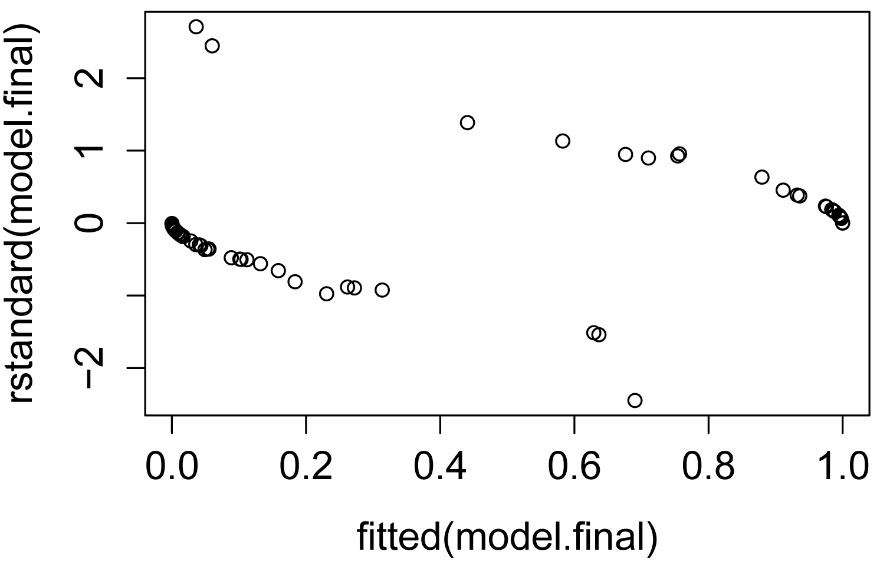简单的预测值图 在最终模型中创建包含变量的数据框，并在NA中省略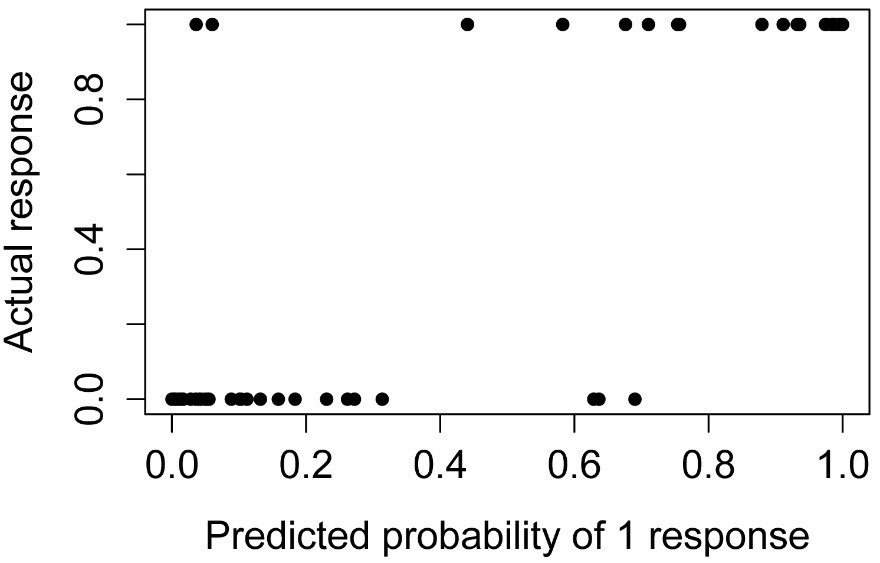过度离散检验 过度离散是glm的deviance残差相对于自由度较大的情况。这些值显示在模型的摘要中。一个指导原则是，如果deviance残差与剩余自由度的比率超过1.5，则模型过度离散。过度离散表明模型不能很好地拟合数据：解释变量可能无法很好地描述因变量，或者可能无法为这些数据正确指定模型。如果存在过度离散，一种可能的解决方案是 在glm中使用quasibinomial family选项。 Null deviance: 93.351 on 69 degrees of freedom Residual deviance: 30.392 on 63 degrees of freedom deviance / df.residual  0.482417 评估模型的替代方法 使用逐步回归程序的替代或补充是将模型与拟合统计进行比较。我的compare.glm 函数将为glm模型显示AIC，AICc，BIC和伪R平方。使用的模型应该都拟合相同的数据。也就是说，如果数据集中的不同变量包含缺失值，则应该谨慎使用。如果您对使用哪种拟合统计数据没有任何偏好，您希望在最终模型中使用较少的项，我可能会推荐AICc或BIC。 一系列模型可以与标准的anova 进行比较。模型应嵌套在先前模型中或anova函数列表中的下一个模型中; 和模型应该拟合相同的数据。在比较多个回归模型时，通常放宽p值为0.10或0.15。 在以下示例中，使用通过逐步回归过程选择的模型。请注意，虽然模型9最小化了AIC和AICc，但模型8最小化了BIC。anova结果表明模型8不是对模型7的显着改进。这些结果支持选择模型7,8或9中的任何一个。 compareGLM(model.1, model.2, model.3, model.4, model.5, model.6, model.7, model.8, model.9)$Models

Formula

1 "Status ~ 1"

2 "Status ~ Release"

3 "Status ~ Release + Upland"

4 "Status ~ Release + Upland + Migr"

5 "Status ~ Release + Upland + Migr + Mass"

6 "Status ~ Release + Upland + Migr + Mass + Indiv"

7 "Status ~ Release + Upland + Migr + Mass + Indiv + Insect"

8 "Status ~ Upland + Migr + Mass + Indiv + Insect"

9 "Status ~ Upland + Migr + Mass + Indiv + Insect + Wood"

\$Fit.criteria

Rank Df.res   AIC  AICc   BIC McFadden Cox.and.Snell Nagelkerke   p.value

1    1     66 94.34 94.53 98.75   0.0000        0.0000     0.0000       Inf

2    2     65 62.13 62.51 68.74   0.3787        0.3999     0.5401 2.538e-09

3    3     64 56.02 56.67 64.84   0.4684        0.4683     0.6325 3.232e-10

4    4     63 51.63 52.61 62.65   0.5392        0.5167     0.6979 7.363e-11

5    5     62 50.64 52.04 63.87   0.5723        0.5377     0.7263 7.672e-11

6    6     61 49.07 50.97 64.50   0.6118        0.5618     0.7588 5.434e-11

7    7     60 46.42 48.90 64.05   0.6633        0.5912     0.7985 2.177e-11

8    6     61 44.71 46.61 60.14   0.6601        0.5894     0.7961 6.885e-12

9    7     60 44.03 46.51 61.67   0.6897        0.6055     0.8178 7.148e-12

Analysis of Deviance Table

Model 1: Status ~ 1

Model 2: Status ~ Release

Model 3: Status ~ Release + Upland

Model 4: Status ~ Release + Upland + Migr

Model 5: Status ~ Release + Upland + Migr + Mass

Model 6: Status ~ Release + Upland + Migr + Mass + Indiv

Model 7: Status ~ Release + Upland + Migr + Mass + Indiv + Insect

Model 8: Status ~ Upland + Migr + Mass + Indiv + Insect

Model 9: Status ~ Upland + Migr + Mass + Indiv + Insect + Wood

Resid. Df Resid. Dev Df Deviance Pr(>Chi)

1        66     90.343

2        65     56.130  1   34.213 4.94e-09 ***

3        64     48.024  1    8.106 0.004412 **

4        63     41.631  1    6.393 0.011458 *

5        62     38.643  1    2.988 0.083872 .

6        61     35.070  1    3.573 0.058721 .

7        60     30.415  1    4.655 0.030970 *

8        61     30.710 -1   -0.295 0.587066

9        60     28.031  1    2.679 0.101686最受欢迎的见解

1.matlab中的偏最小二乘回归（PLSR）和主成分回归（PCR）

2.R语言多元Logistic逻辑回归 应用案例

3.R语言面板平滑转移回归(PSTR)分析案例实现

4.R语言回归中的Hosmer-Lemeshow拟合优度检验

5.R语言泊松Poisson回归模型分析案例

6.r语言中对LASSO回归，Ridge岭回归和Elastic Net模型实现

7.R语言如何在生存分析与Cox回归中计算IDI，NRI指标

8.在R语言中实现Logistic逻辑回归

9.R语言实现向量自回归VAR模型


展开全文R语言 应用案例
• 多元Logistic_回归分析多元Logistic_回归分析
• 多元 logistic 回归 1. 下面是子宫内膜癌的病例对照研究数据暴露因素是雌激素 组 使用过雌激素 未使用过雌激素 病例组 55 a 128 b 对照组 19 c 164 d 问题使用过雌激素是否是子宫内膜癌的危险因素危险强度为多少 2. ...
• 如何用spss17.0进行二元和多元logistic回归分析
• 1. softmax函数 2. 与二元Logistic回归的关系 3. 误差函数 3.1 多元回归的1-of-K表示 3.2 训练样本集的似然函数 3.3 交叉熵误差函数 4. 最大似然估计 代码实现（mnist数据集）
Multi-class Logistic Regression1. softmax函数2. 与二元Logistic回归的关系3. 误差函数3.1 多元回归的1-of-K表示3.2 训练样本集的似然函数3.3 交叉熵误差函数4. 最大似然估计代码实现（mnist数据集）
$\qquad$在 $\text{Logistic\ Regression}$摘记 一文中对二元$\text{Logistic}$回归进行了详细的介绍，本文主要描述采用 $\text{softmax}$ 函数实现多元$\text{Logistic}$回归。
1. softmax函数
$\qquad$对于某个输入 $\boldsymbol x$，其对应的 $\text{softmax}$ 输出为向量值 $\boldsymbol y=[y_1,\cdots,y_k,\cdots,y_K]^T$，且满足 $\sum\limits_{k=1}^K y_k=1$。
$\qquad(1)$ 分类问题 $(K>2)$ 中使用 $\text{softmax}$ 函数表示输出值分量：
$\qquad\qquad$ $y_k=\dfrac{e^{a_k}}{\sum\limits_{j=1}^K e^{a_j}}=\dfrac{e^{\boldsymbol{w}_k^{T}\boldsymbol{x}+b_k}}{\sum\limits_{j=1}^K e^{\boldsymbol{w}_j^{T}\boldsymbol{x}+b_j}}\ ,\ k=1,2,\cdots,K$
$\qquad(2)$ 对于多元Logistic回归：　$a_j=\boldsymbol{w}_j^{T}\boldsymbol{x}+b_j$
$\qquad$　　其中，$\boldsymbol{x}\in R^d,\boldsymbol{w}_j=[w_{j,1},w_{j,2},\cdots,w_{j,d}]^T\ \ (j=1,2,\cdots,K)$
$\qquad$
$\qquad$　　若记 $\boldsymbol{w}_j^*=[\boldsymbol{w}_j^T,b_j]^T$ 和 $\boldsymbol{x}^*=[\boldsymbol{x}^T,1]^T$，则 $a_j=\boldsymbol{w}_j^{T}\boldsymbol{x}+b_j={(\boldsymbol{w}_j^*)}^{T}\boldsymbol{x}^*$
$\qquad$
$\qquad$　 【为了方便描述】可以略掉 ‘$*$’ 号，直接写成：　 $a_j=\boldsymbol{w}_j^{T}\boldsymbol{x}$
$\qquad$
$\qquad(3)$ 将输出值分量 $y_k$ 描述成后验概率的形式：
$\qquad\qquad$ $y_k=p(y=k|\boldsymbol x)=\dfrac{e^{\boldsymbol{w}_k^{T}\boldsymbol{x}}}{\sum\limits_{j=1}^K e^{\boldsymbol{w}_j^{T}\boldsymbol{x}}}\ ,\ k=1,2,\cdots,K$
$\qquad$
2. 与二元Logistic回归的关系

对比二元Logistic回归

$\boldsymbol{x}$为正例的概率：$p(y=1|\boldsymbol{x})=\dfrac{1}{1+e^{-(\boldsymbol{w}^{T}\boldsymbol{x}+b)}}$
$\boldsymbol{x}$为负例的概率：$p(y=0|\boldsymbol{x})=1-\dfrac{1}{1+e^{-(\boldsymbol{w}^{T}\boldsymbol{x}+b)}}=\dfrac{e^{-(\boldsymbol{w}^{T}\boldsymbol{x}+b)}}{1+e^{(\boldsymbol{w}^{T}\boldsymbol{x}+b)}}=\dfrac{1}{1+e^{-(\boldsymbol{w}^{T}\boldsymbol{x}+b)}}$

$\qquad$当 $K=2$ 时，$\text{softmax}$函数实际上等同于二元$\text{Logistic}$回归（假设 $k=\{0,1\}$）：
$\qquad\qquad$ $\begin{cases} \ \ \ y_0=p(y=0|\boldsymbol x)=\dfrac{e^{\boldsymbol{w}_0^{T}\boldsymbol{x}}}{e^{\boldsymbol{w}_0^{T}\boldsymbol{x}}+e^{\boldsymbol{w}_1^{T}\boldsymbol{x}}}=\dfrac{1}{1+e^{(\boldsymbol{w}_1-\boldsymbol{w}_0)^{T}\boldsymbol{x}}}\\ \\ \ \ \ y_1=p(y=1|\boldsymbol x)=\dfrac{e^{\boldsymbol{w}_1^{T}\boldsymbol{x}}}{e^{\boldsymbol{w}_0^{T}\boldsymbol{x}}+e^{\boldsymbol{w}_1^{T}\boldsymbol{x}}}=\dfrac{1}{1+e^{-(\boldsymbol{w}_1-\boldsymbol{w}_0)^{T}\boldsymbol{x}}} \end{cases}$
$\qquad$令 $\hat\boldsymbol{w}=\boldsymbol{w}_1-\boldsymbol{w}_0$，那么类后验概率就是二元$\text{Logistic}$回归中情形。
$\qquad$
3. 误差函数
$\qquad$针对多元Logistic回归，首先要写出其误差函数。
$\qquad$假设训练样本集为 $\{ (\boldsymbol{x}_n,c_n)\} _{n=1}^{N}$，其中 $\boldsymbol{x}_n\in R^{d},c_n\in \{1,2,\cdots,K\}$，参数为 $\boldsymbol W=(\boldsymbol{w}_1^T,\boldsymbol{w}_2^T,\cdots,\boldsymbol{w}_K^T,b)^T$。

二元Logistic回归
假设训练样本为 $\{ (\boldsymbol{x}_n,c_n)\} _{n=1}^{N}$，其中 $\boldsymbol{x}_n\in R^{d},c_n\in \{0,1\}$，似然函数为：

$\qquad\qquad L(\boldsymbol{w},b)= \displaystyle\prod_{n=1}^N h(\boldsymbol{x}_n)^{c_n}\left[ 1-h(\boldsymbol{x}_n)\right] ^{1-c_n}\ ,\ h(\boldsymbol{x})=p(c=1|\boldsymbol{x})=\dfrac{1}{1+e^{-(\boldsymbol{w}^{T}\boldsymbol{x}+b)}}$

取“负的对数似然函数”作为误差函数，即：$l(\boldsymbol{w},b)=-\ln L(\boldsymbol{w},b)$。

3.1 多元回归的1-of-K表示
$\qquad(1)$ 用变量 $c\in \{1,2,\cdots,K\}$ 表示输入 $\boldsymbol x$ 所对应的类别
$\qquad(2)$ 引入目标向量 $\bold t=[0,\cdots,0,1,0,\cdots,0]^T\in R^K$，满足 $t_k=1,t_j=0\ (j\neq k)$
$\qquad$　　表示“输入 $\boldsymbol x$ 属于第 $k$ 类” 或者说变量 $c=k$
$\qquad(3)$ 用向量值 $\boldsymbol y=[y_1,\cdots,y_k,\cdots,y_K]^T$ 表示输入 $\boldsymbol x$ 所对应的 $\textbf{softmax}$输出
$\qquad$　　$y_k=p(c=k|\boldsymbol x)=p(t_k=1|\boldsymbol x)=\displaystyle\prod_{k=1}^K p(t_k|\boldsymbol x)^{t_k}=\dfrac{e^{\boldsymbol{w}_k^{T}\boldsymbol{x}}}{\sum\limits_{j=1}^K e^{\boldsymbol{w}_j^{T}\boldsymbol{x}}}$
$\qquad$　　显然，$\sum\limits_{k=1}^K y_k=\sum\limits_{k=1}^K p(y=k|\boldsymbol x)=1$
$\qquad$
3.2 训练样本集的似然函数
$\qquad(1)$ 对于第 $n$ 个训练样本 $(\boldsymbol{x}_n,c_n)$，其 $\text{softmax}$ 输出为 $\boldsymbol y_n=[y_{n1},\cdots,y_{nk},\cdots,y_{nK}]^T$，且
$\qquad\qquad\ y_{nk}=p(c_n=k|\boldsymbol x_n)=p(t_{nk}=1|\boldsymbol x_n)=\displaystyle\prod_{k=1}^K p(t_{nk}|\boldsymbol x_n)^{t_{nk}}$
$\qquad(2)$ 训练样本集 $\{ (\boldsymbol{x}_n,c_n)\} _{n=1}^{N}$ 的似然函数 $L(\boldsymbol W)$ 为：
\qquad\qquad\ \begin{aligned}L(\boldsymbol W)&= \displaystyle\prod_{n=1}^N p(c_n=k|\boldsymbol x_n) \\ &=\displaystyle\prod_{n=1}^N p(t_{nk}=1|\boldsymbol x_n) \\ &=\displaystyle\prod_{n=1}^N \displaystyle\prod_{k=1}^K p(t_{nk}|\boldsymbol x_n)^{t_{nk}} \\ \end{aligned}
$\qquad$
3.3 交叉熵误差函数
$\qquad$取“负的对数似然函数”为（交叉熵）误差函数 $\text{(cross-entropy\ error\ function)}$
\qquad\qquad\begin{aligned} l(\boldsymbol W)&=-\ln L(\boldsymbol W)=-\displaystyle\sum_{n=1}^N \displaystyle\sum_{k=1}^K t_{nk}\ln p(t_{nk}|\boldsymbol x_n)\\ &=-\displaystyle\sum_{n=1}^N \displaystyle\sum_{k=1}^K t_{nk}\ln y_{nk}\end{aligned}
$\qquad$使用交叉熵作为误差函数，是因为：
$\qquad(1)$ 若训练样本 $\boldsymbol x_n$ 的类别 $c_n=k$，则对应的目标向量 $\bold t_n$ 只有第 $k$ 个分量 $t_{nk}=1$，而其他分量 $t_{nj}=0\ (j\neq k)$。
$\qquad(2)$ 在训练过程中，$y_{nk}$ 是训练样本 $\boldsymbol x_n$ 所对应 $\text{softmax}$ 输出的第 $k$ 个分量（训练样本的正确类别 $k$ 所对应的输出分量值）。
$\qquad(3)$ 如果正确类别 $k$ 所对应分量值 $y_{nk}$ 越大，$\ln y_{nk}$ 也越大，交叉熵就越小，训练误差也就越小。
$\qquad(4)$ 理想情况下，正确类别 $k$ 所对应分量值 $y_{nk}=1,\forall\ n$，那么交叉熵为 $0$，也就是没有训练误差。
$\qquad$
4. 最大似然估计
$\qquad$为了求出参数 $\boldsymbol W=(\boldsymbol{w}_1^T,\boldsymbol{w}_2^T,\cdots,\boldsymbol{w}_K^T,b)^T$，同样采用最大似然估计。
$\qquad$可以将训练样本集分成 $K$ 个子集 $C_1,\cdots,C_k,\cdots,C_K$，第 $k$ 个子集 $C_k$ 中的所有样本 $\boldsymbol x_n$ 的类别都为 $c_n=k$，对应的目标向量 $\bold t_n$ 都满足 $t_{nk}=1,t_{nj}=0\ (j\neq k)$，由误差函数的表达式：
\qquad\qquad\begin{aligned} l(\boldsymbol W)&=-\displaystyle\sum_{n=1}^N \displaystyle\sum_{k=1}^K t_{nk}\ln y_{nk}\\ &=-\displaystyle\sum_{n\in C_1} t_{n1}\ln y_{n1}-\cdots-\displaystyle\sum_{n\in C_k} t_{nk} \ln y_{nk}-\cdots-\displaystyle\sum_{n\in C_K} t_{nK}\ln y_{nK} \\ &=-\displaystyle\sum_{n\in C_1} \ln y_{n1}-\cdots-\displaystyle\sum_{n\in C_k} \ln y_{nk}-\cdots-\displaystyle\sum_{n\in C_K} \ln y_{nK} \end{aligned}
$\qquad$对 $l(\boldsymbol W)$ 求参数 $\boldsymbol w_k$ 的偏导分为两个部分：
$\qquad$
$\qquad(1)$ 对 $l(\boldsymbol W)$ 的第 $k$ 个分量 $l_k(\boldsymbol W)=-\displaystyle\sum_{n\in C_k} \ln y_{nk}$ 求参数 $\boldsymbol w_k$ 的偏导
\qquad\qquad\begin{aligned} \dfrac{\partial l_k(\boldsymbol W)}{\partial \boldsymbol w_k}&=-\displaystyle\sum_{n\in C_k} \dfrac{1}{ y_{nk}} \dfrac{\partial y_{nk}}{\partial \boldsymbol w_k}\qquad\qquad (\boldsymbol{w}_k^{T}\boldsymbol{x}_n=\boldsymbol{x}_n^{T}\boldsymbol{w}_k)\\ &=-\displaystyle\sum_{n\in C_k} \dfrac{1}{ y_{nk}} \dfrac{(e^{\boldsymbol{w}_k^{T}\boldsymbol{x}_n})^{'}\sum\limits_{j=1}^K e^{\boldsymbol{w}_j^{T}\boldsymbol{x}_n}-e^{\boldsymbol{w}_k^{T}\boldsymbol{x}_n}\left(\sum\limits_{j=1}^K e^{\boldsymbol{w}_j^{T}\boldsymbol{x}_n} \right)^{'}}{\left(\sum\limits_{j=1}^K e^{\boldsymbol{w}_j^{T}\boldsymbol{x}_n}\right)^2}\\ &=-\displaystyle\sum_{n\in C_k} \dfrac{1}{ y_{nk}} \dfrac{(e^{\boldsymbol{w}_k^{T}\boldsymbol{x}_n})\boldsymbol{x}_n\sum\limits_{j=1}^K e^{\boldsymbol{w}_j^{T}\boldsymbol{x}_n}-e^{\boldsymbol{w}_k^{T}\boldsymbol{x}_n}(e^{\boldsymbol{w}_k^{T}\boldsymbol{x}_n})\boldsymbol{x}_n}{\left(\sum\limits_{j=1}^K e^{\boldsymbol{w}_j^{T}\boldsymbol{x}_n}\right)^2}\\ &=-\displaystyle\sum_{n\in C_k} \dfrac{1}{ y_{nk}} \dfrac{(e^{\boldsymbol{w}_k^{T}\boldsymbol{x}_n})\boldsymbol{x}_n}{\sum\limits_{j=1}^K e^{\boldsymbol{w}_j^{T}\boldsymbol{x}_n}}\dfrac{\sum\limits_{j=1}^K e^{\boldsymbol{w}_j^{T}\boldsymbol{x}_n}-e^{\boldsymbol{w}_k^{T}\boldsymbol{x}_n}}{\sum\limits_{j=1}^K e^{\boldsymbol{w}_j^{T}\boldsymbol{x}_n}}\\ &=-\displaystyle\sum_{n\in C_k} \dfrac{1}{ y_{nk}} y_{nk}\boldsymbol{x}_n(1-y_{nk})\\ &=-\displaystyle\sum_{n\in C_k}(1-y_{nk})\boldsymbol{x}_n \\ \end{aligned}
$\qquad(2)$ 对 $l(\boldsymbol W)$ 的第 $i\ (i\neq k)$ 个分量 $l_i(\boldsymbol W)=-\displaystyle\sum_{n\in C_i} \ln y_{ni}$ 求参数 $\boldsymbol w_k$ 的偏导
\qquad\qquad\begin{aligned} \dfrac{\partial l_i(\boldsymbol W)}{\partial \boldsymbol w_k}&=-\displaystyle\sum_{n\in C_i} \dfrac{1}{ y_{ni}} \dfrac{\partial y_{ni}}{\partial \boldsymbol w_k}\\ &=-\displaystyle\sum_{n\in C_i} \dfrac{1}{ y_{ni}} \dfrac{(e^{\boldsymbol{w}_i^{T}\boldsymbol{x}_n})^{'}\sum\limits_{j=1}^K e^{\boldsymbol{w}_j^{T}\boldsymbol{x}_n}-e^{\boldsymbol{w}_i^{T}\boldsymbol{x}_n}\left(\sum\limits_{j=1}^K e^{\boldsymbol{w}_j^{T}\boldsymbol{x}_n} \right)^{'}}{\left(\sum\limits_{j=1}^K e^{\boldsymbol{w}_j^{T}\boldsymbol{x}_n}\right)^2}\\ &=-\displaystyle\sum_{n\in C_i} \dfrac{1}{ y_{ni}} \dfrac{-e^{\boldsymbol{w}_i^{T}\boldsymbol{x}_n}(e^{\boldsymbol{w}_k^{T}\boldsymbol{x}_n})\boldsymbol{x}_n}{\left(\sum\limits_{j=1}^K e^{\boldsymbol{w}_j^{T}\boldsymbol{x}_n}\right)^2}\\ &=-\displaystyle\sum_{n\in C_i} \dfrac{1}{ y_{ni}} \dfrac{e^{\boldsymbol{w}_i^{T}\boldsymbol{x}_n}}{\sum\limits_{j=1}^K e^{\boldsymbol{w}_j^{T}\boldsymbol{x}_n}}\dfrac{-(e^{\boldsymbol{w}_k^{T}\boldsymbol{x}_n})\boldsymbol{x}_n}{\sum\limits_{j=1}^K e^{\boldsymbol{w}_j^{T}\boldsymbol{x}_n}}\\ &=-\displaystyle\sum_{n\in C_i} \dfrac{1}{ y_{ik}} y_{ni}(-y_{nk})\boldsymbol{x}_n\\ &=-\displaystyle\sum_{n\in C_i}(-y_{nk})\boldsymbol{x}_n \\ \end{aligned}
$\qquad$综合起来，两个公式可以表示为：
$\qquad\qquad\ \dfrac{\partial l(\boldsymbol W)}{\partial \boldsymbol w_k}=-\displaystyle\sum_{n=1}^N (t_{nk}-y_{nk})\boldsymbol{x}_n$
$\qquad$采用梯度下降法时，权值更新公式为：
$\qquad\qquad\ \boldsymbol{W}^{(m+1)}=\boldsymbol{W}^{(m)}-\alpha\dfrac{\partial l(\boldsymbol W)}{\partial \boldsymbol W}$
$\qquad$其中 $\alpha$ 为梯度下降法的步长。
$\qquad$
代码实现（mnist数据集）
import numpy as np
from dataset.mnist import load_mnist

def softmax_train(train,target,alpha,num):
xhat = np.concatenate((train,np.ones((len(train),1))),axis=1)
nparam = len(xhat.T) #785
beta = np.random.rand(nparam,10)    #785x10
for i in range(num):
wtx = np.dot(xhat,beta)
wtx1 = wtx - np.max(wtx,axis=1).reshape(len(train),1)
e_wtx = np.exp(wtx1)
yx = e_wtx/np.sum(e_wtx,axis=1).reshape(len(xhat),1)
print('  #'+str(i+1)+' : '+str(cross_entropy(yx,target)))
t1 = target - yx
t2 = np.dot(xhat.T, t1)
beta = beta + alpha*t2

return beta

def cross_entropy(yx,t):
sum1 = np.sum(yx*t,axis=1)
ewx = np.log(sum1+0.000001)
return -np.sum(ewx)/len(yx)

def classification(test, beta, test_t):
xhat = np.concatenate((test,np.ones((len(test),1))),axis=1)
wtx = np.dot(xhat,beta)
output = np.where(wtx==np.max(wtx,axis=1).reshape((len(test),1)))

print("Percentage Correct: ",np.where(output==test_t).shape/len(test))
return np.array(output,dtype=np.uint8)

if __name__ == '__main__':
(x_train, t_train), (x_test, t_test) = load_mnist(flatten=True, normalize=False)

nread = 60000
train_in = x_train[:nread,:]
train_tgt = np.zeros((nread,10))

test_in = x_test[:10000,:]
test_t = t_test[:10000]

for i in range(nread):
train_tgt[i,t_train[i]] = 1

beta = softmax_train(train_in,train_tgt,0.001,60)
print(beta)
result = classification(test_in, beta, test_t)

测试结果：
#1 : 5.626381119337011
#2 : 5.415158063701459
#3 : 10.959830171565791
#4 : 8.062787294189338
#5 : 7.4643357380759765
#6 : 9.070059164063883
#7 : 9.81079287953052
#8 : 7.13921201579068
#9 : 7.176904417794094
#10 : 4.607102717465571
#11 : 3.9215536116316625
#12 : 4.199011112147004
#13 : 4.135313269465135
#14 : 3.214738972020379
#15 : 2.804664146283606
#16 : 2.901161881757491
#17 : 2.9996749271603456
#18 : 2.609904566490558
#19 : 2.6169338357951197
#20 : 2.538795429964946
#21 : 2.7159497447897256
#22 : 2.634980803678192
#23 : 2.974848646434367
#24 : 3.1286179795674154
#25 : 3.2208869228881407
#26 : 2.548910343301664
#27 : 2.5298981152704743
#28 : 2.3826001247525035
#29 : 2.4498572463653243
#30 : 2.3521370651353837
#31 : 2.4309032741212664
#32 : 2.366133209606206
#33 : 2.4462922376053364
#34 : 2.3850487760328933
#35 : 2.4481429887352792
#36 : 2.370067560256672
#37 : 2.376729198498193
#38 : 2.297488373847759
#39 : 2.265126273640295
#40 : 2.258495714414137
#41 : 2.327524884607823
#42 : 2.3130200962416128
#43 : 2.290046983208286
#44 : 2.1465196716967805
#45 : 2.0969060851949677
#46 : 1.8901858209971119
#47 : 1.844354795879705
#48 : 1.6340799726564934
#49 : 1.60064459794013
#50 : 1.4667008762515674
#51 : 1.4453938385590863
#52 : 1.3767004735390218
#53 : 1.359619935503484
#54 : 1.3153462460865966
#55 : 1.309895715988472
#56 : 1.2799649790773286
#57 : 1.2807586745656392
#58 : 1.2559139323742572
#59 : 1.2582212637839076
#60 : 1.237819660093416
权值：
[[7.69666472e-01 2.16009202e-01 9.81729719e-01 … 5.32453082e-01
7.88719040e-01 5.14326954e-01]
[3.90401951e-01 5.84040914e-01 7.94883641e-01 … 8.02009249e-01
3.29345264e-02 6.70861290e-01]
[8.69075434e-02 8.43381782e-01 4.77683466e-01 … 8.71965798e-01
4.47018470e-04 5.07498017e-01]
…
[7.96129468e-01 6.14364951e-01 8.32783158e-01 … 6.53493763e-01
2.06235991e-01 8.60469591e-01]
[1.67070291e-01 3.23211147e-02 2.41519794e-01 … 6.56026583e-01
5.98396521e-01 5.42304452e-01]
[8.43299673e-01 6.22843596e-01 6.05652099e-02 … 1.10339403e-01
1.61855811e-01 3.29385438e-01]]
识别率：
Percentage Correct:  0.9037


展开全文• 介绍多元回归和logistic回归的原理，并介绍spss的具体操作，对学习有帮助
• 什么是线性回归 有监督学习 => 学习样本为D={(xi,yi)}i=1ND=\{(x_i,y_i)\}^N_{i=1}D={(xi​,yi​)}i=1N​ 输出/预测的结果yiy_iyi​为连续变量 需要学习映射f:x→yf:x...多元线性回归 损失函数（loss function...
什么是线性回归

有监督学习 => 学习样本为$D=\{(x_i,y_i)\}^N_{i=1}$
输出/预测的结果$y_i$为连续变量
需要学习映射$f:x→y$
假定输入x与输出y之间有线性相关关系

一元线性回归
$y=ax+b$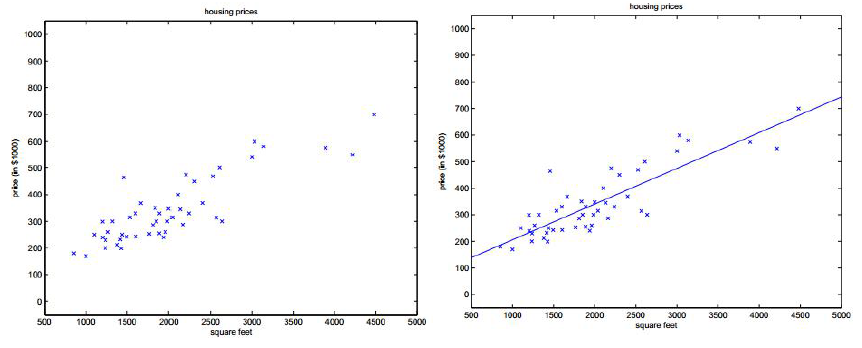多元线性回归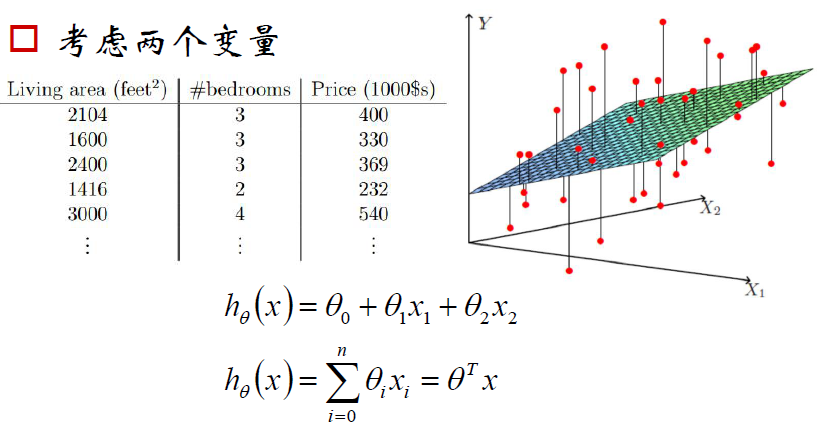损失函数（loss function）
我们要找到最好的权重/参数$[\theta_0,\theta_1,...,\theta_n]=\theta$
我们把x到y的映射函数f记作$\theta$的函数$h_\theta(x)$
定义损失函数为: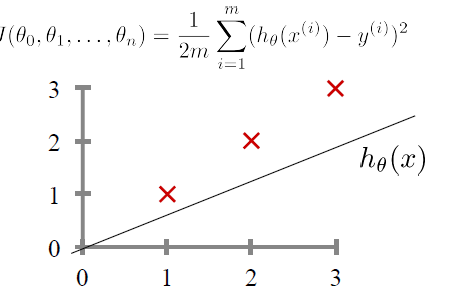梯度下降
逐步最小化损失函数的过程
如同下山，找准方向(梯度)，每次迈进一小步，直至山底（注：这里的$\frac{1}{2m}$只是一个系数，加上去是只是为了计算方便，下面的笔记中可能有些地方会省去，请勿在意）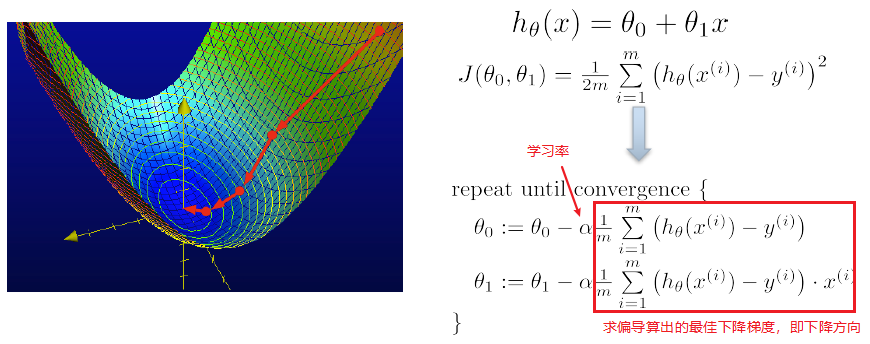在坐标系中的表示方法如下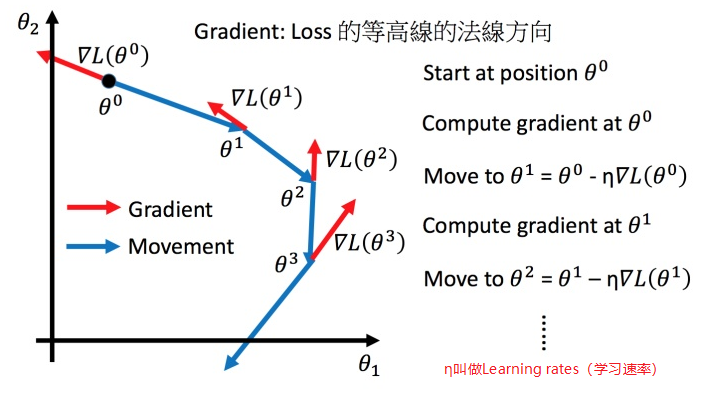假如现在有n个特征/变量$x_j(j=1...n)$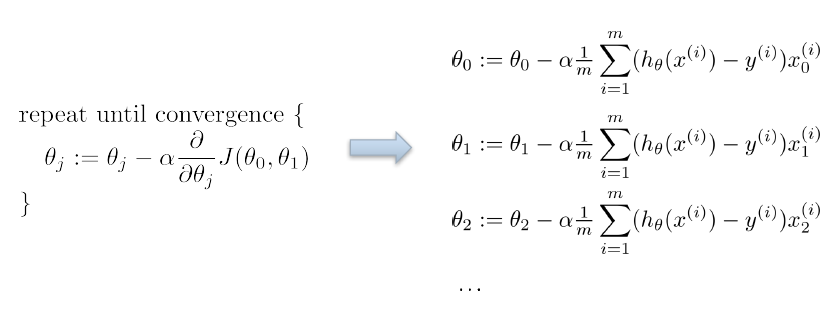Tip1：调整学习速率
小心翼翼地调整学习率
举例：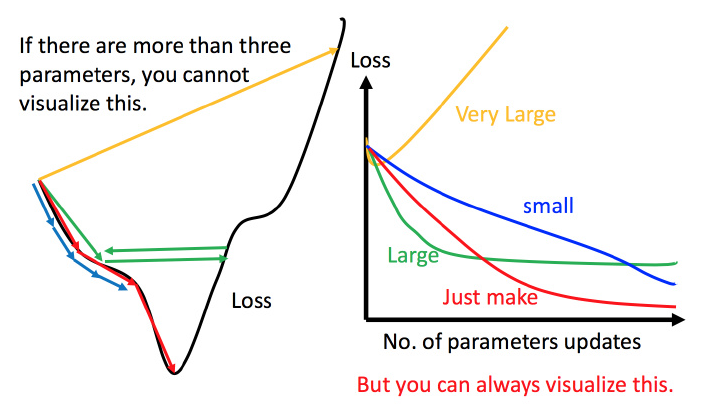上图左边黑色为损失函数的曲线，假设从左边最高点开始，如果学习率调整的刚刚好，比如红色的线，就能顺利找到最低点。如果学习率调整的太小，比如蓝色的线，就会走的太慢，虽然这种情况给足够多的时间也可以找到最低点，实际情况可能会等不及出结果。如果 学习率调整的有点大，比如绿色的线，就会在上面震荡，走不下去，永远无法到达最低点。还有可能非常大，比如黄色的线，直接就飞出去了，更新参数的时候只会发现损失函数越更新越大。
虽然这样的可视化可以很直观观察，但可视化也只是能在参数是一维或者二维的时候进行，更高维的情况已经无法可视化了。
解决方法就是上图右边的方案，将参数改变对损失函数的影响进行可视化。比如学习率太小（蓝色的线），损失函数下降的非常慢；学习率太大（绿色的线），损失函数下降很快，但马上就卡住不下降了；学习率特别大（黄色的线），损失函数就飞出去了；红色的就是差不多刚好，可以得到一个好的结果。
自适应学习率
举一个简单的思想：随着次数的增加，通过一些因子来减少学习率

通常刚开始，初始点会距离最低点比较远，所以使用大一点的学习率

update好几次参数之后呢，比较靠近最低点了，此时减少学习率

比如 $\eta^t =\frac{\eta^t}{\sqrt{t+1}}$，$t$ 是次数。随着次数的增加，$\eta^t$ 减小

学习率不能是一个值通用所有特征，不同的参数需要不同的学习率
Adagrad 算法
每个参数的学习率都把它除上之前微分的均方根。解释：
普通的梯度下降为：
$w^{t+1} \leftarrow w^t -η^tg^t$
$\eta^t =\frac{\eta^t}{\sqrt{t+1}}$

$w$ 是一个参数

Adagrad 可以做的更好：
$w^{t+1} \leftarrow w^t -\frac{η^t}{\sigma^t}g^t$
$g^t =\frac{\partial L(\theta^t)}{\partial w}$

$\sigma^t$ :之前参数的所有微分的均方根，对于每个参数都是不一样的。

Adagrad举例
下图是一个参数的更新过程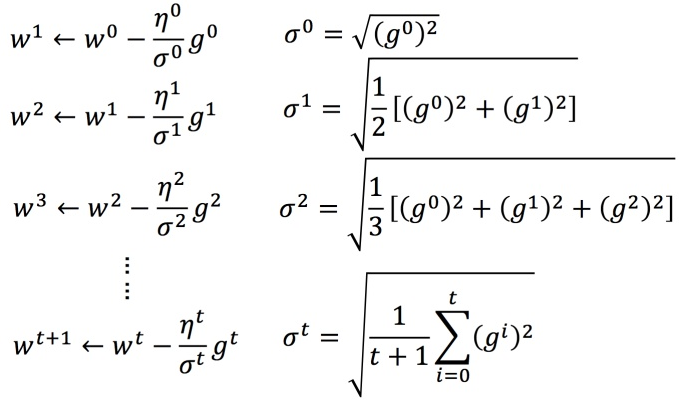将 Adagrad 的式子进行化简：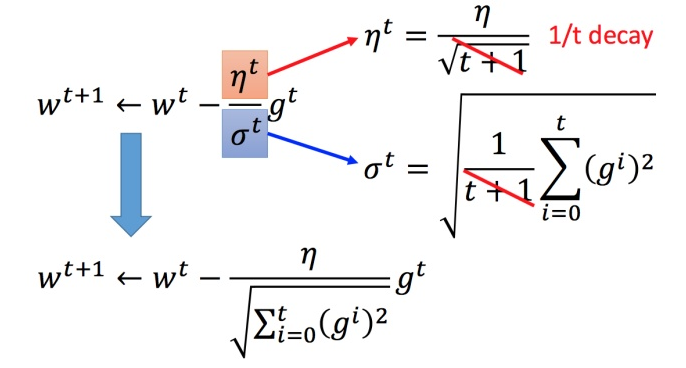Adagrad 存在的矛盾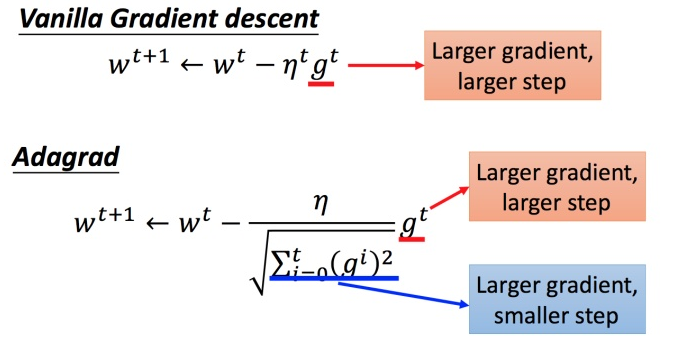在 Adagrad 中，当梯度越大的时候，步伐应该越大，但下面分母又导致当梯度越大的时候，步伐会越小。
下图是一个直观的解释：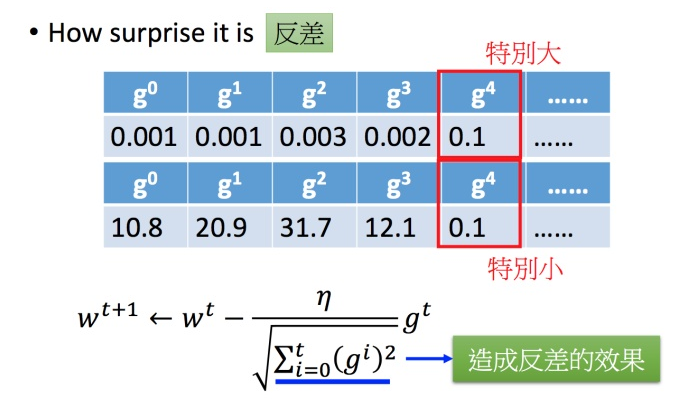下面给一个正式的解释：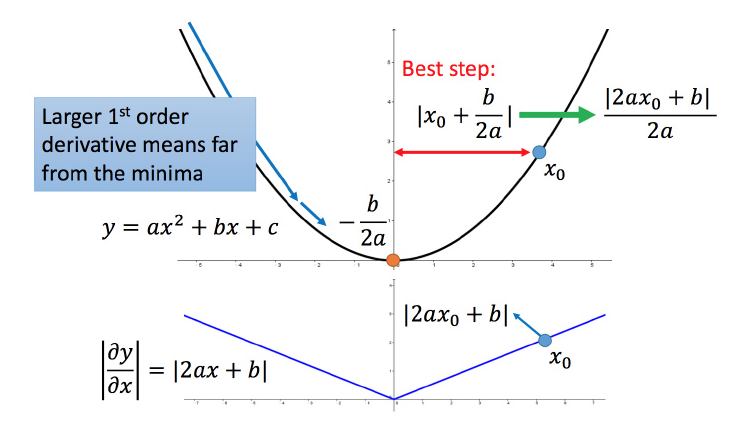比如初始点在 $x_0$，最低点为 $−\frac{b}{2a}$，最佳的步伐就是 $x0$ 到最低点之间的距离 $\left | x_0+\frac{b}{2a} \right |$，也可以写成 $\left | \frac{2ax_0+b}{2a} \right |$。而刚好 $|2ax_0+b|$ 就是方程绝对值在 $x_0$ 这一点的微分。
这样可以认为如果算出来的微分越大，则距离最低点越远。而且最好的步伐和微分的大小成正比。所以如果踏出去的步伐和微分成正比，它可能是比较好的。
结论：梯度越大，就跟最低点的距离越远。
这个结论在多个参数的时候就不一定成立了。
多参数下结论不一定成立
对比不同的参数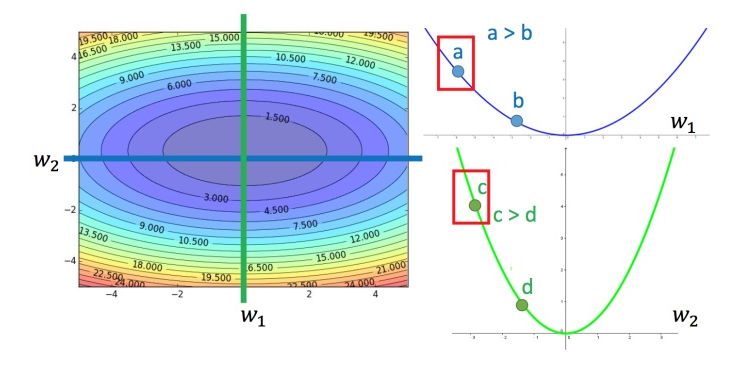上图左边是两个参数的损失函数，颜色代表损失函数的值。如果只考虑参数 $w_1$，就像图中蓝色的线，得到右边上图结果；如果只考虑参数 $w_2$，就像图中绿色的线，得到右边下图的结果。确实对于 $a$ 和 $b$，结论是成立的，同理 $c$ 和 $b$ 也成立。但是如果对比$a$ 和 $c$，就不成立了，$c$ 比 $a$ 大，但 $c$ 距离最低点是比较近的。
所以上述结论是在没有考虑跨参数对比的情况下，才能成立的。所以还不完善。
之前说到的最佳距离 $\left | \frac{2ax_0+b}{2a} \right |$，还有个分母 $2a$ 。对function进行二次微分刚好可以得到:$\frac{\partial ^2y}{\partial x^2} = 2a$
所以最好的步伐应该是：$\frac{一次微分}{二次微分}$
即不止和一次微分成正比，还和二次微分成反比。最好的step应该考虑到二次微分：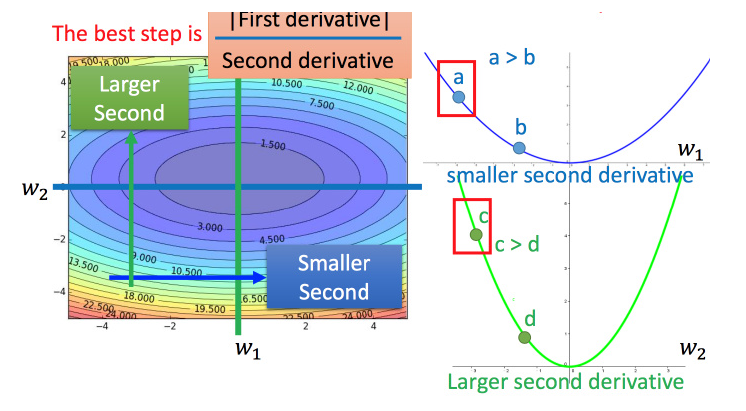Adagrad 进一步的解释
再回到之前的 Adagrad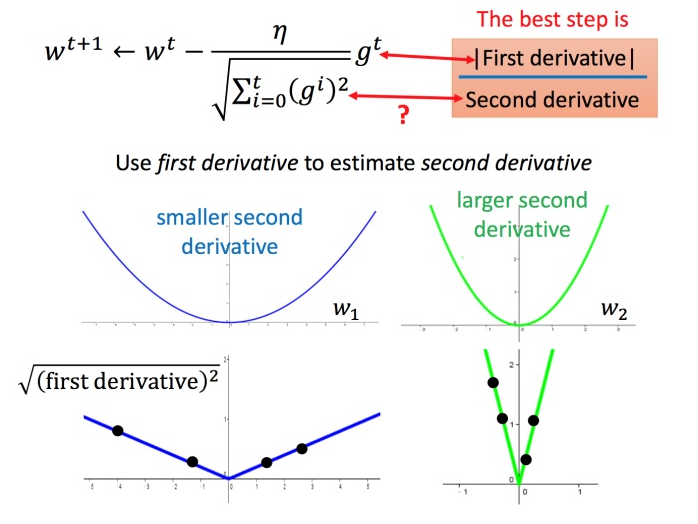对于 $\sqrt{\sum_{i=0}^t(g^i)^2}$ 就是希望再尽可能不增加过多运算的情况下模拟二次微分。（如果计算二次微分，在实际情况中可能会增加很多的时间消耗）
Tip2：随机梯度下降法
之前的梯度下降：
$L=\sum_n(\hat y^n-(b+\sum w_ix_i^n))^2$
$\theta^i =\theta^{i-1}- \eta\triangledown L(\theta^{i-1})$
而随机梯度下降法更快：
损失函数不需要处理训练集所有的数据，选取一个例子 $x^n$
$L=(\hat y^n-(b+\sum w_ix_i^n))^2$
$\theta^i =\theta^{i-1}- \eta\triangledown L^n(\theta^{i-1})$
此时不需要像之前那样对所有的数据进行处理，只需要计算某一个例子的损失函数$L_n$，就可以赶紧更新梯度。
对比：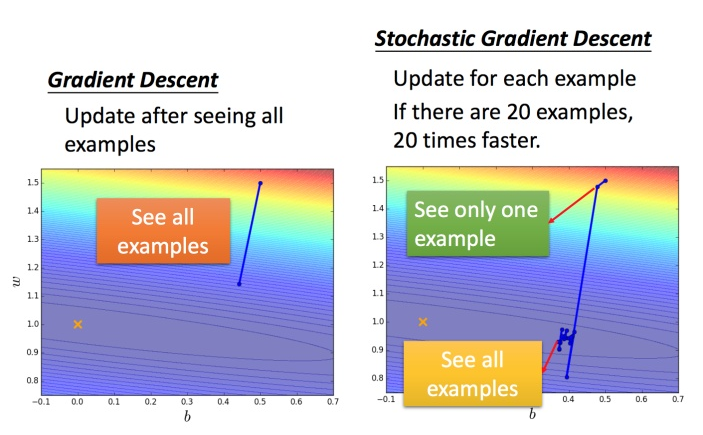常规梯度下降法走一步要处理到所有二十个例子，但随机算法此时已经走了二十步（每处理一个例子就更新）
Tip3：mini-batch
不是每拿到一个样本即更改梯度，而是若干个样本的平均梯度作为更新方向，则是mini-batch梯度下降算法。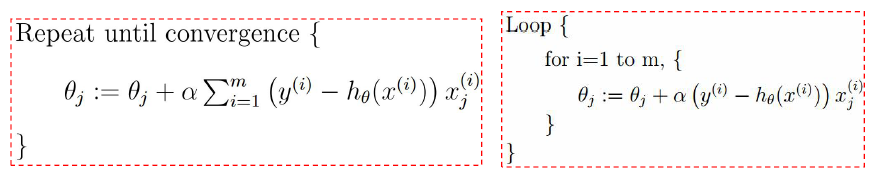Tip4：特征缩放
比如有个函数：
$y=b+w_1x_1+w_2x_2 \tag{12}$
两个输入的分布的范围很不一样，建议把他们的范围缩放，使得不同输入的范围是一样的。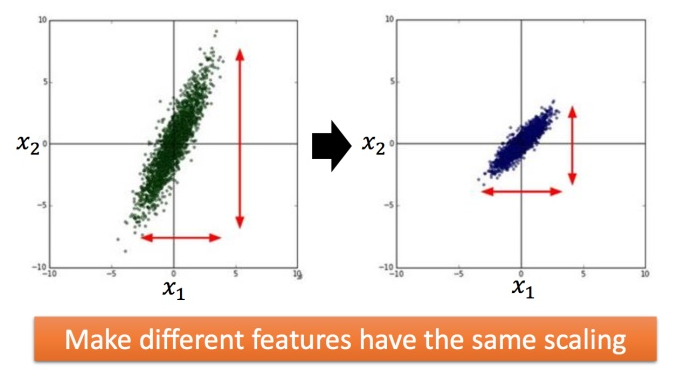为什么要这样做？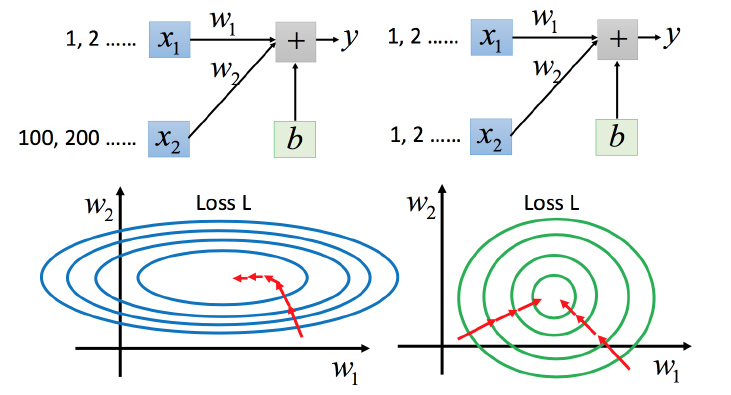上图左边是 $x_1$ 的规模比 $x_2$ 要小很多，所以当 $w_1$ 和 $w_2$ 做同样的变化时，$w_1$ 对 $y$ 的变化影响是比较小的，$x_2$ 对 $y$ 的变化影响是比较大的。
坐标系中是两个参数的error surface（现在考虑左边蓝色），因为 $w_1$ 对 $y$ 的变化影响比较小，所以 $w_1$ 对损失函数的影响比较小，$w_1$ 对损失函数有比较小的微分，所以 $w_1$ 方向上是比较平滑的。同理 $x_2$ 对 $y$ 的影响比较大，所以 $x_2$ 对损失函数的影响比较大，所以在 $x_2$ 方向有比较尖的峡谷。
上图右边是两个参数规模比较接近，右边的绿色图就比较接近圆形。
对于左边的情况，上面讲过这种狭长的情形不过不用Adagrad的话是比较难处理的，两个方向上需要不同的学习率，同一组学习率会搞不定它。而右边情形更新参数就会变得比较容易。左边的梯度下降并不是向着最低点方向走的，而是顺着等高线切线法线方向走的。但绿色就可以向着圆心（最低点）走，这样做参数更新也是比较有效率。
怎么做缩放？
方法非常多，这里举例一种常见的做法：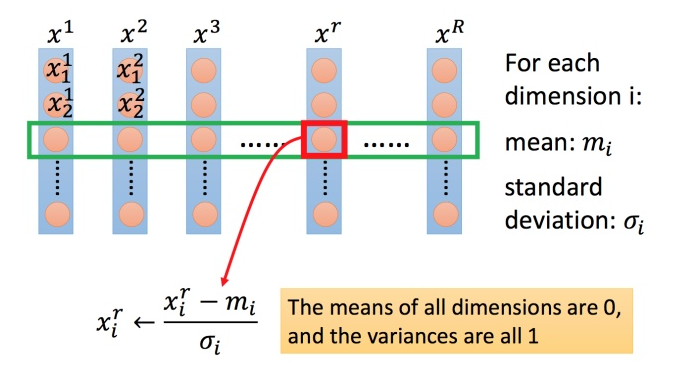上图每一列都是一个例子，里面都有一组特征。
对每一个维度 $i$（绿色框）都计算平均数，记做 $m_i$；还要计算标准差，记做 $\sigma _i$。
然后用第 $r$ 个例子中的第 $i$ 个输入，减掉平均数 $m_i$，然后除以标准差 $\sigma _i$，得到的结果是所有的维数都是 $0$，所有的方差都是 $1$
underfitting vs overfitting
回归与欠/过拟合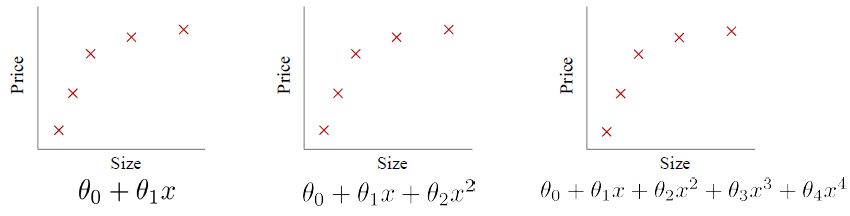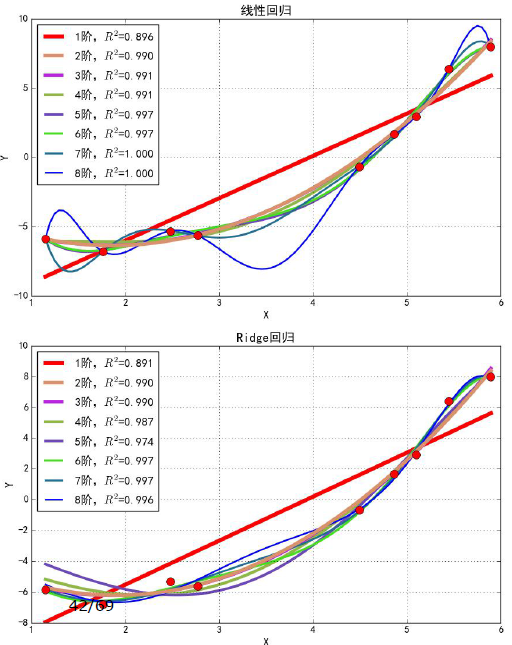过拟合问题：如果我们有非常多特征/模型很复杂, 我们的假设函数曲线可以对原始数据拟合得非常好), 但丧失了一般性，从而导致对新给的待预测样本，预测效果差.
Coefficient of Determination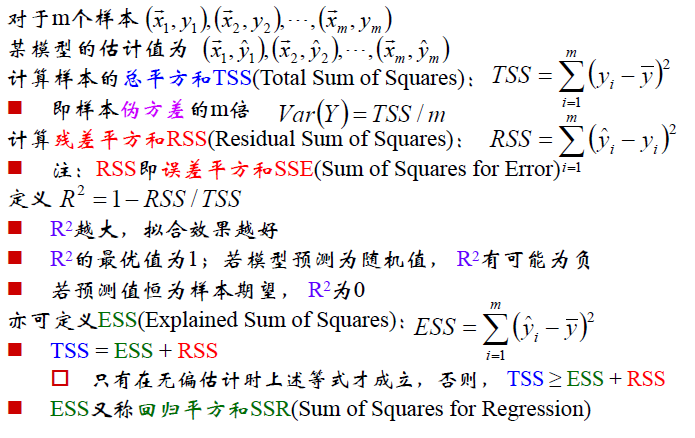正则化

控制参数幅度，不让模型“无法无天”
限制参数搜索空间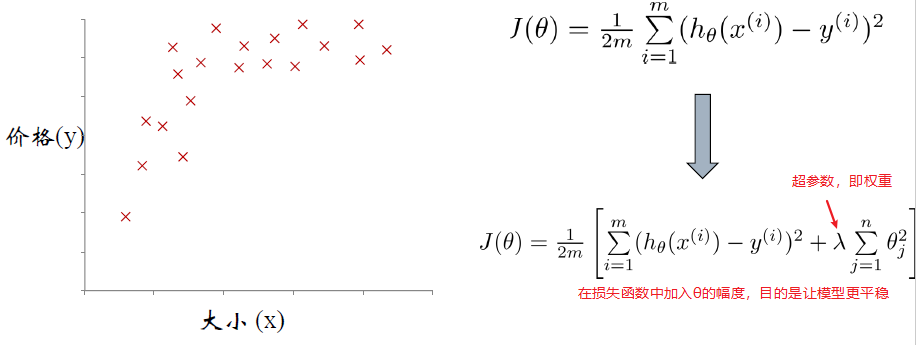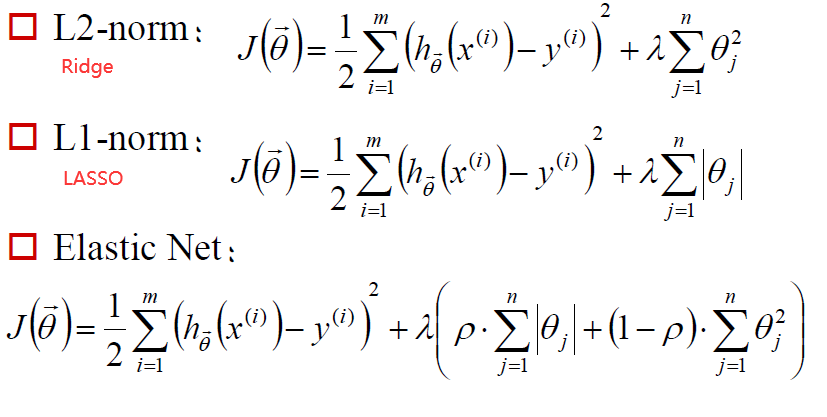岭回归和lasso回归
岭回归(Ridge regression: Hoerl and Kennard, 1970) 的原理和OLS 估计类似，但是对系数的大小设置了惩罚项。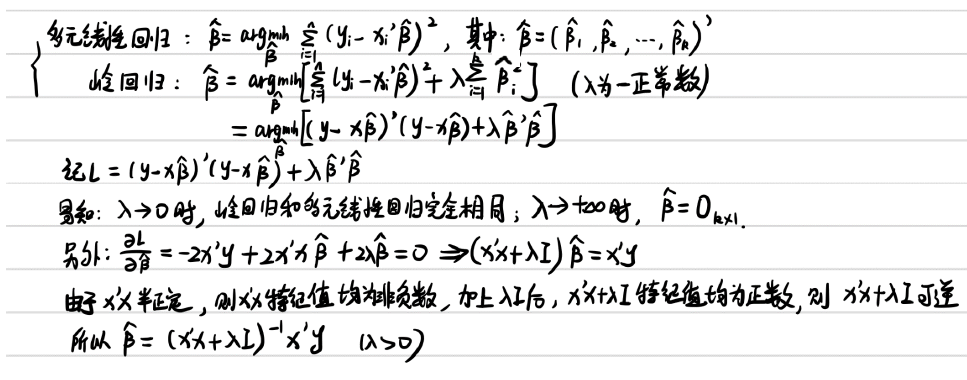lasso回归的原理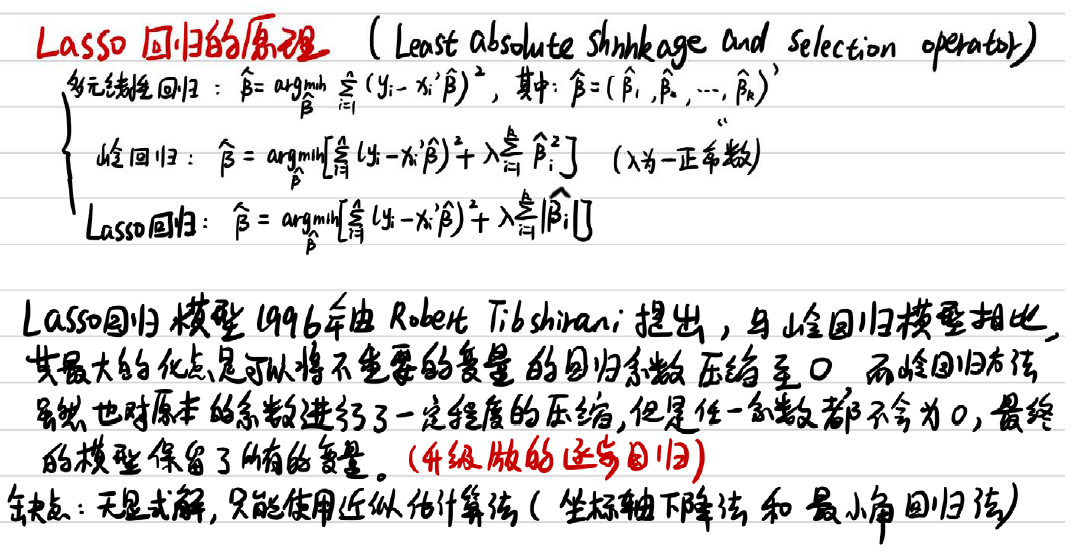Logistic回归
logistic适用于解决分类问题，通过使用sigmoid函数完成由值到概率的转变，从而完成分类任务
线性回归-Logistic回归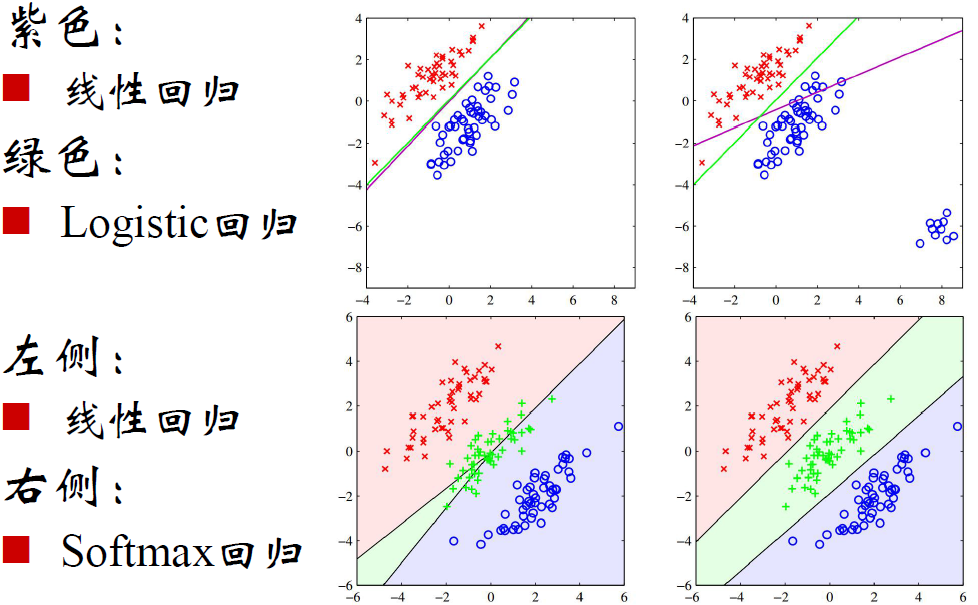sigmoid函数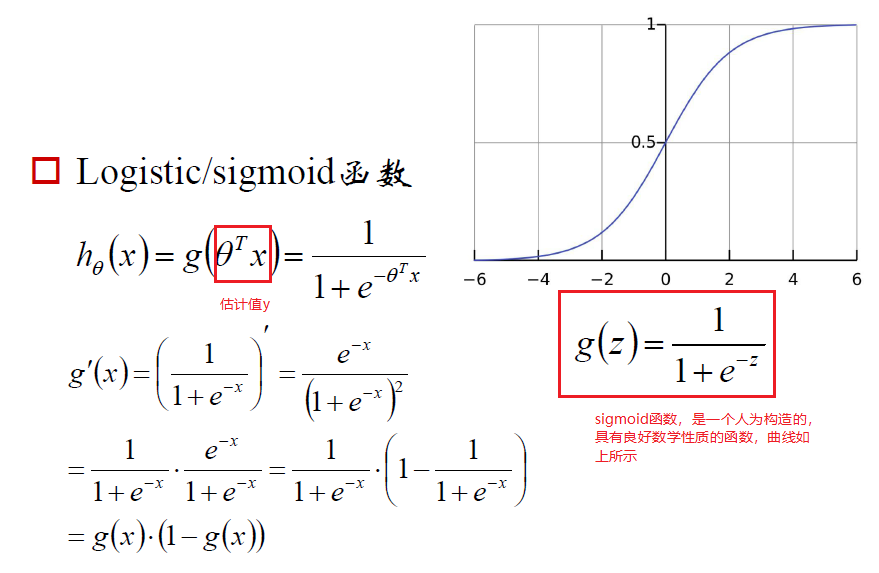Logistic回归参数估计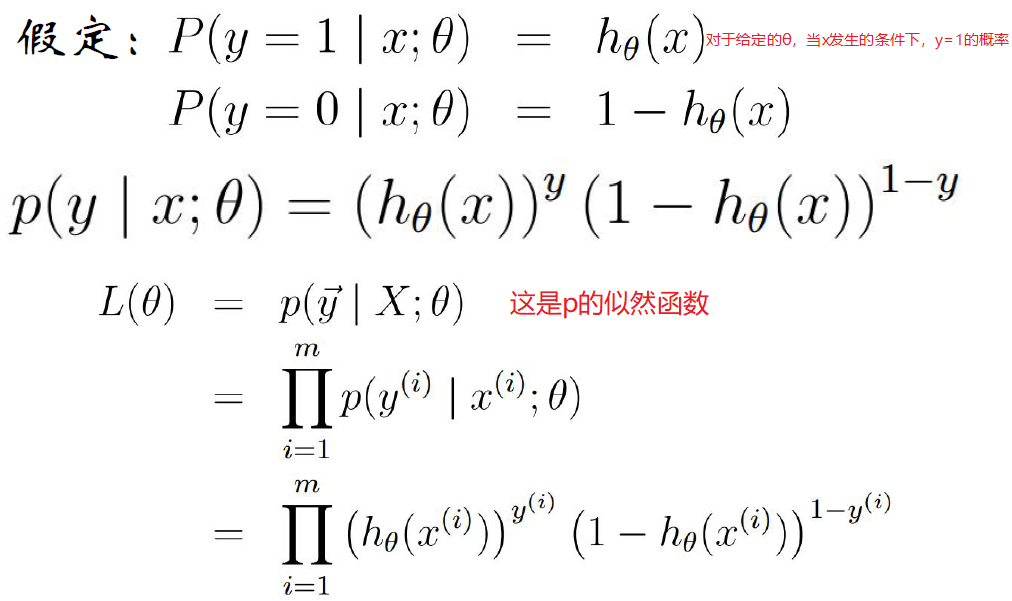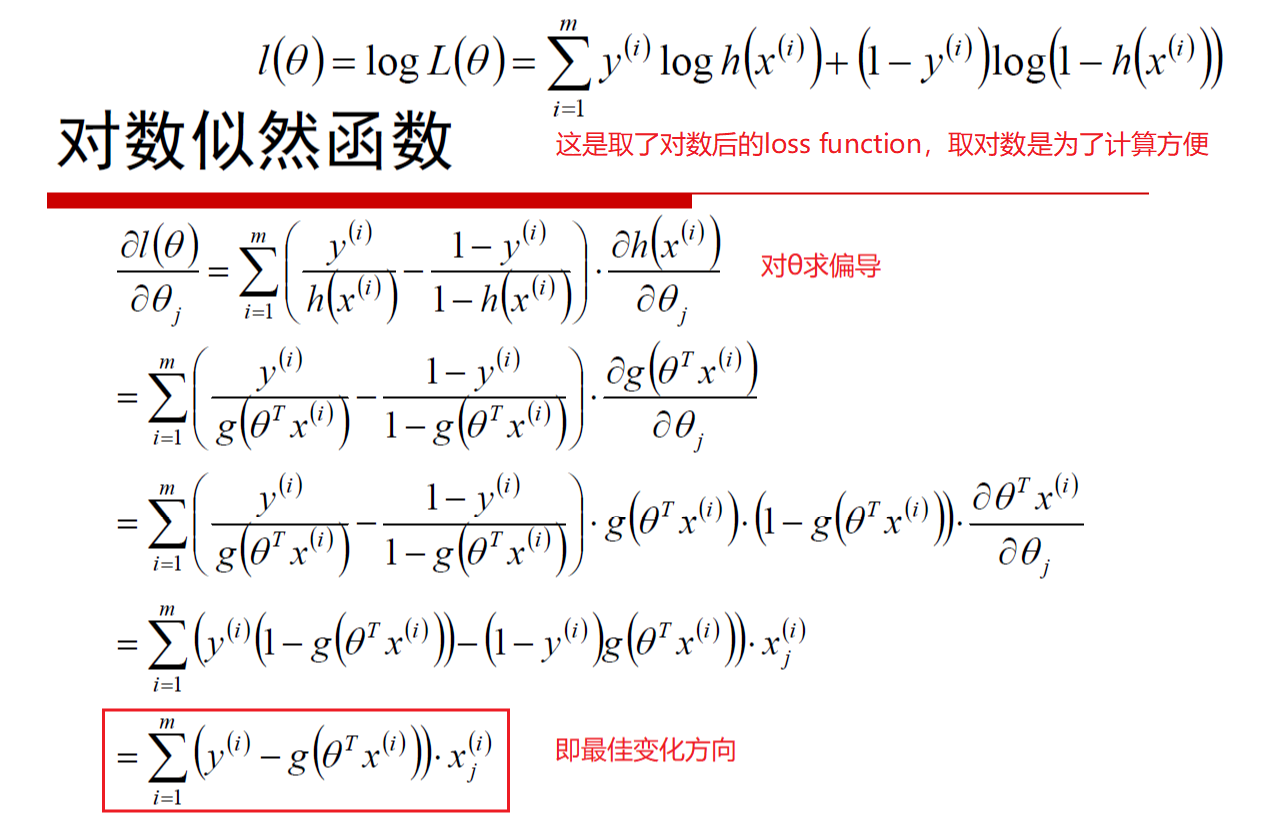Logistic回归参数的学习规则为：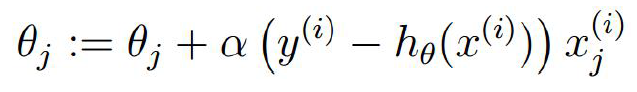然后接下来的步骤和回归就一样了，防止过拟合的方法也是一样，例如在损失函数中加上惩罚因子后的样子如下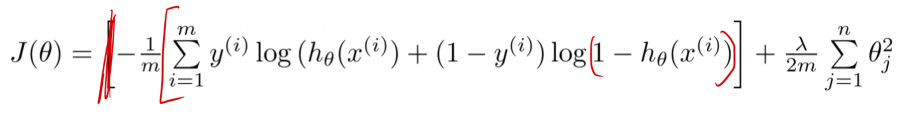展开全文• 在这里讲述如何通过MATLAB的代码实现多元logistic回归模型，对于想用MATLAB来实现多元logistic回归模型的朋友有很大的帮助。
• ## 二元Logistic回归

千次阅读 2020-10-16 22:58:08
多分类无序：多元Logistic回归 多分类有序：定序回归 (ordinal regression) 按个案是否经过匹配分类 非条件Logistic回归 条件Logistic回归 二元Logistic回归概述 一般线性模型中的一种，即反应变量 (dependent...
引言
搞了若干次这个东西，还是似懂非懂。得啃啃这个骨头了。
概述

研究分类反应变量与多个因素之间的关系，使用Logistic回归

典型例子：疾病的病因 （危险因素）分析

按反应变量分类

二分类：二元Logistic回归
多分类无序：多元Logistic回归
多分类有序：定序回归 (ordinal regression)

按个案是否经过匹配分类

非条件Logistic回归
条件Logistic回归

二元Logistic回归概述
一般线性模型中的一种，即反应变量 (dependent variables)为二分类变量的回归分析，模型输出为变量取特定值的概率。

反应变量为二分类 （某事件发生/不发生）：使用二元Logistic回归

e.g. 患病-不患病，死亡-生存
二分类后的反应变量必须覆盖全集 （即必须为两种可能之一，不可能有第三种）

应用：预测某事件发生的概率，筛选某事件发生的危险因素
其他类似但不可使用二元Logistic回归的情形

既关心某事件发生/不发生，也关心该事件何时发生：使用生存分析 (survival analysis)
反应变量为多分类变量：使用多元逻辑斯蒂回归
反应变量为等级变量：使用定序回归 (ordinal regression)

注意事项

对样本量要求较高
欲筛选的危险因素/预测指标越多，研究所需的样本量越大
因素较多时，交叉分类数量将很大，需要每个分类下有足够的样本量，以保证参数估计的稳定性
重视对自变量的取舍

保证每一分类下有足够的样本量
最好应能结合具体专业知识及流行病学意义对自变量的影响予以阐释
调整自变量通常会进行多次

Logistic模型
以Y表示二分类反应变量，量化赋值：Y=1（阳性）；Y=0（阴性）。
拟考察的自变量假设有m个，记为$X_1,X_2,...X_m$。
设$P(Y=1)=P$。
称下面模型为Logistic模型：
$P=\frac {e^{(\beta_0+\beta_1X_1+\beta_2X_2+...+\beta_mX_m)}}{1+e^{(\beta_0+\beta_1X_1+\beta_2X_2+...+\beta_mX_m)}}$
其中，$\beta_0$称为常数项/截距，$\beta_i$称为自变量$X_i$的回归系数。
当$e^{(\beta_0+\beta_1X_1+\beta_2X_2+...+\beta_mX_m)}$在${(-\infty,+\infty)}$之间变动时，$P$在0到1之间变动。
对P取Logit变换，可将该模型线性化：
$logit(P)=ln\frac{P}{1-P}=\beta_0+\beta_1X_1+\beta_2X_2+...+\beta_mX_m$
如此可以估算诸参数，并分析反应变量和自变量之间的关系
自变量
当自变量为数值变量时：直接纳入模型即可；当自变量为二分类、多分类无序、多分类有序变量时，需对变量进行赋值。
多分类有序变量

当有理由认为该有序变量反映了某种距离关系时，可以赋值为相应的表示距离的数值

e.g. 尿蛋白水平-,+,++,+++分别赋值为1，10，100，1000

当变量不同水平间缺乏明确可评价的距离关系时，作为无序变量处理

多分类无序变量
采用哑变量 (dummy variable)赋值方法。

对于可取k个不同水平的变量$X_i$
定义(k-1)个变量$X_{i1},X_{i2},...X_{i{(k-1)}}$

使得变量取第j (j≤k-1)个水平等价于$“X_{ij}=1，其余X_{ir}=0”$
变量取第k个水平等价于$“\forall j \in \{1,2,...,k-1\}, X_{ij}=0”$

称$X_{i1},X_{i2},...X_{i{(k-1)}}$为表征$X_i$的哑变量

二分类无序变量
在保证变量两水平下赋值相对大小不变的情况下，不同的赋值方式，参数估计、假设检验将得到一样的结果。
例如：对于双水平二分类变量$X_i$，下列两种赋值方式将会得到相同的统计结果：
$\begin{cases} X_1=1\\ X_2=0\\ \end{cases}$
与
$\begin{cases} X_1=2\\ X_2=1\\ \end{cases}$
实务
在R中运行二元Logistic回归。
参考材料

《医学统计学》八年制，第二版


展开全文统计学 R
• ## logit和logistic模型

万次阅读 2016-10-31 21:35:01
关于logit和logistic模型的区别貌似是个老生常谈的问题，学习之后稍微整理一下： ...（2）应用上，普通logistic的响应变量是二元的，多元logistic的因变量可为多元。logit的响应变量可以是多元的。 （3）统计软广义线性模型
• ## logistic回归学习

千次阅读 2013-12-30 16:11:54
因变量为二分类的称为二项logistic回归，因变量为多分类的称为多元logistic回归。因变量的类型: 可为连续变量、等级变量、分类变量。适用性 两元因变量的logistic回归模型方程讲解 一个自变量与Y
• 基于分步Logistic回归的太阳黑子与武汉降雨量研究，马英钧，陈楚，由于采用累积多元Logistic回归建立的武汉降雨量与太阳黑子的关系模型，得到的效果并不理想。本文在分步Logistic回归-
• matlab用多元非线性规划，求logistic函数因变量的最大值，自变量有3个，都是维数为809的向量，其中两个自变量的具体值已给出。 目标函数： function p=fun1(x) [A]=xlsread('********.xls'); w=A(:,1); ...c++ c语言
• 如何用spss17.0进行二元和多元logistic回归分析 一、二元logistic回归分析 二元logistic回归分析的前提为因变量是可以转化为0、1的二分变量，如：死亡或者生存，男性或者女性，有或无，Yes或No，是或否的情况。 ...
• ## logistic回归

千次阅读 2018-10-28 19:04:43
值得一提的是，logistic回归跟多元线性回归有很多相似之处，模型形式也都是wx+b，w、b为要求的参数，不同点在于因变量不同，logistic回归通过logistic函数L将wx+b对应一个隐形状态p，即p=L（wx+b），若L是logistic...
• 一、 概述 Logistic回归主要用于因变量为分类变量（如疾病的缓解、不缓解，...因变量为二分类的称为二项logistic回归，因变量为多分类的称为多元logistic回归。  下面学习一下Odds、OR、RR的概念： 在病例
• import numpy as np import pandas as pd import scipy.optimize as opt import matplotlib.pyplot as plt plt.rcParams['font.sans-serif']=['SimHei'] #显示中文标签 plt.rcParams['axes.unicode_minus']=False ...python
• 多元线性方程： sigmoid非线性激活函数： 假设函数：，这里假设表示预测为正例的概率，则w为预测为负例的概率。则最后整合表示为,表示预测为真实值的概率。值越大越好。 损失函数：似然函数为，对数似然函数：，...
• LogisticRegression 多元逻辑斯蒂回归，并实现随机梯度下降和L1/L2正则化项。 参照 在此基础上加入L1和L2 Regularization；关于逻辑斯蒂回归中的L1和L2正则化项详见以下两个链接: 并对输入格式进行泛化，例如可以对...C++
• 结合实际情况，可以将Logistic回归分析分为3类，分别是二元Logistic回归分析、多元有序Logistic回归分析和多元无序Logistic回归分析，如下图。 SPSSAU Logistic回归分析分类 Logistic回归分析用于研究X对Y的...数据分析
• 一、Multiclass Classification: One-vs-all（多元分类：一对多） （1）下图左边是二元分类的图形，右边是三元分类的图形（代表多元分类）。在二元分类中，我们可以划出一条拟合曲线（直线、曲线、圆或者椭圆等），...机器学习
• ## logistic回归模型

千次阅读 多人点赞 2020-04-13 17:44:45
从这一期开始，我们准备介绍一系列机器学习算法模型，主要包括logistic回归，决策树，随机森林，关联规则，朴素贝叶斯，支持向量机模型，隐式马尔可夫模型，因子分析，主成分分析，聚类，多元线性回归，时间序列，...损失函数 适用范围
• 该文档讲述了 多元线性回归模型以及logistic模型的研究...